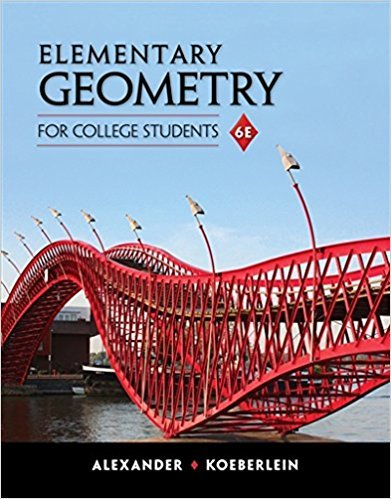×
×

# Solutions for Chapter 11: Introduction to Trigonometry## Full solutions for Elementary Geometry for College Students | 6th Edition

ISBN: 9781285195698Solutions for Chapter 11: Introduction to Trigonometry

Solutions for Chapter 11
4 5 0 338 Reviews
29
0
##### ISBN: 9781285195698

Elementary Geometry for College Students was written by and is associated to the ISBN: 9781285195698. This textbook survival guide was created for the textbook: Elementary Geometry for College Students, edition: 6. Chapter 11: Introduction to Trigonometry includes 47 full step-by-step solutions. Since 47 problems in chapter 11: Introduction to Trigonometry have been answered, more than 3153 students have viewed full step-by-step solutions from this chapter. This expansive textbook survival guide covers the following chapters and their solutions.

Key Math Terms and definitions covered in this textbook
• Affine transformation

Tv = Av + Vo = linear transformation plus shift.

• Back substitution.

Upper triangular systems are solved in reverse order Xn to Xl.

• Cayley-Hamilton Theorem.

peA) = det(A - AI) has peA) = zero matrix.

• Complete solution x = x p + Xn to Ax = b.

(Particular x p) + (x n in nullspace).

A sequence of steps (end of Chapter 9) to solve positive definite Ax = b by minimizing !x T Ax - x Tb over growing Krylov subspaces.

• Diagonal matrix D.

dij = 0 if i #- j. Block-diagonal: zero outside square blocks Du.

• Dimension of vector space

dim(V) = number of vectors in any basis for V.

• Exponential eAt = I + At + (At)2 12! + ...

has derivative AeAt; eAt u(O) solves u' = Au.

• Fourier matrix F.

Entries Fjk = e21Cijk/n give orthogonal columns FT F = nI. Then y = Fe is the (inverse) Discrete Fourier Transform Y j = L cke21Cijk/n.

• Fundamental Theorem.

The nullspace N (A) and row space C (AT) are orthogonal complements in Rn(perpendicular from Ax = 0 with dimensions rand n - r). Applied to AT, the column space C(A) is the orthogonal complement of N(AT) in Rm.

• Hankel matrix H.

Constant along each antidiagonal; hij depends on i + j.

• Krylov subspace Kj(A, b).

The subspace spanned by b, Ab, ... , Aj-Ib. Numerical methods approximate A -I b by x j with residual b - Ax j in this subspace. A good basis for K j requires only multiplication by A at each step.

• Multiplication Ax

= Xl (column 1) + ... + xn(column n) = combination of columns.

• Normal equation AT Ax = ATb.

Gives the least squares solution to Ax = b if A has full rank n (independent columns). The equation says that (columns of A)·(b - Ax) = o.

• Orthogonal matrix Q.

Square matrix with orthonormal columns, so QT = Q-l. Preserves length and angles, IIQxll = IIxll and (QX)T(Qy) = xTy. AlllAI = 1, with orthogonal eigenvectors. Examples: Rotation, reflection, permutation.

• Positive definite matrix A.

Symmetric matrix with positive eigenvalues and positive pivots. Definition: x T Ax > 0 unless x = O. Then A = LDLT with diag(D» O.

• Right inverse A+.

If A has full row rank m, then A+ = AT(AAT)-l has AA+ = 1m.

• Simplex method for linear programming.

The minimum cost vector x * is found by moving from comer to lower cost comer along the edges of the feasible set (where the constraints Ax = b and x > 0 are satisfied). Minimum cost at a comer!

• Unitary matrix UH = U T = U-I.

Orthonormal columns (complex analog of Q).

• Volume of box.

The rows (or the columns) of A generate a box with volume I det(A) I.

×